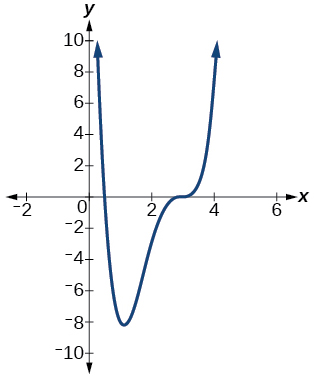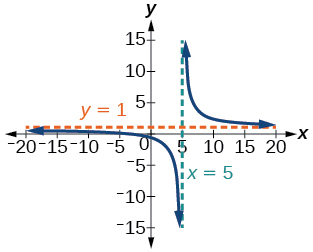$$\newcommand{\id}{\mathrm{id}}$$ $$\newcommand{\Span}{\mathrm{span}}$$ $$\newcommand{\kernel}{\mathrm{null}\,}$$ $$\newcommand{\range}{\mathrm{range}\,}$$ $$\newcommand{\RealPart}{\mathrm{Re}}$$ $$\newcommand{\ImaginaryPart}{\mathrm{Im}}$$ $$\newcommand{\Argument}{\mathrm{Arg}}$$ $$\newcommand{\norm}{\| #1 \|}$$ $$\newcommand{\inner}{\langle #1, #2 \rangle}$$ $$\newcommand{\Span}{\mathrm{span}}$$

# 3.R: Polynomial and Rational Functions(Review)

•• Contributed by Jay Abramson
• Principal Lecturer (School of Mathematical and Statistical Sciences) at Arizona State University
• Publisher: OpenStax CNX
$$\newcommand{\vecs}{\overset { \rightharpoonup} {\mathbf{#1}} }$$ $$\newcommand{\vecd}{\overset{-\!-\!\rightharpoonup}{\vphantom{a}\smash {#1}}}$$$$\newcommand{\id}{\mathrm{id}}$$ $$\newcommand{\Span}{\mathrm{span}}$$ $$\newcommand{\kernel}{\mathrm{null}\,}$$ $$\newcommand{\range}{\mathrm{range}\,}$$ $$\newcommand{\RealPart}{\mathrm{Re}}$$ $$\newcommand{\ImaginaryPart}{\mathrm{Im}}$$ $$\newcommand{\Argument}{\mathrm{Arg}}$$ $$\newcommand{\norm}{\| #1 \|}$$ $$\newcommand{\inner}{\langle #1, #2 \rangle}$$ $$\newcommand{\Span}{\mathrm{span}}$$ $$\newcommand{\id}{\mathrm{id}}$$ $$\newcommand{\Span}{\mathrm{span}}$$ $$\newcommand{\kernel}{\mathrm{null}\,}$$ $$\newcommand{\range}{\mathrm{range}\,}$$ $$\newcommand{\RealPart}{\mathrm{Re}}$$ $$\newcommand{\ImaginaryPart}{\mathrm{Im}}$$ $$\newcommand{\Argument}{\mathrm{Arg}}$$ $$\newcommand{\norm}{\| #1 \|}$$ $$\newcommand{\inner}{\langle #1, #2 \rangle}$$ $$\newcommand{\Span}{\mathrm{span}}$$

## 3.1 Complex Numbers

Perform the indicated operation with complex numbers.

1) $$(4+3 i)+(-2-5 i)$$

$$2-2 i$$

2) $$(6-5 i)-(10+3 i)$$

3) $$(2-3 i)(3+6 i)$$

$$24+3 i$$

4) $$\dfrac{2-i}{2+i}$$

Solve the following equations over the complex number system.

5) $$x^{2}-4 x+5=0$$

$$\{2+i, 2-i\}$$

6) $$x^{2}+2 x+10=0$$

For the exercises 1-2, write the quadratic function in standard form. Then, give the vertex and axes intercepts. Finally, graph the function.

1) $$f(x)=x^{2}-4 x-5$$

$$f(x)=(x-2)^{2}-9$$ vertex $$(2,-9)$$, intercepts $$(5,0); (-1,0); (0,-5)$$2) $$f(x)=-2 x^{2}-4 x$$

For the problems 3-4, find the equation of the quadratic function using the given information.

3) The vertex is $$(-2,3)$$ and a point on the graph is $$(3,6)$$.

$$f(x)=\dfrac{3}{25}(x+2)^{2}+3$$

4) The vertex is $$(-3,6.5)$$ and a point on the graph is $$(2,6)$$.

5) A rectangular plot of land is to be enclosed by fencing. One side is along a river and so needs no fence. If the total fencing available is $$600$$ meters, find the dimensions of the plot to have maximum area.

$$300$$ meters by $$150$$ meters, the longer side parallel to river.

6) An object projected from the ground at a $$45$$ degree angle with initial velocity of $$120$$ feet per second has height, $$h$$, in terms of horizontal distance traveled, $$x$$, given by $$h(x)=\dfrac{-32}{(120)^{2}} x^{2}+x$$. Find the maximum height the object attains.

## 3.3 Power Functions and Polynomial Functions

For the exercises 1-3, determine if the function is a polynomial function and, if so, give the degree and leading coefficient.

1) $$f(x)=4 x^{5}-3 x^{3}+2 x-1$$

Yes, $$\text{degree} = 5$$, $$\text{leading coefficient} = 4$$

2) $$f(x)=5^{x+1}-x^{2}$$

3) $$f(x)=x^{2}\left(3-6 x+x^{2}\right)$$

Yes, $$\text{degree} = 4$$, $$\text{leading coefficient} = 1$$

For the exercises 4-6, determine end behavior of the polynomial function.

4) $$f(x)=2 x^{4}+3 x^{3}-5 x^{2}+7$$

5) $$f(x)=4 x^{3}-6 x^{2}+2$$

As $$x \rightarrow-\infty, f(x) \rightarrow-\infty$$, as $$x \rightarrow \infty, f(x) \rightarrow \infty$$

6) $$f(x)=2 x^{2}\left(1+3 x-x^{2}\right)$$

## 3.4 Graphs of Polynomial Functions

For the exercises 1-3, find all zeros of the polynomial function, noting multiplicities.

1) $$f(x)=(x+3)^{2}(2 x-1)(x+1)^{3}$$

$$-3$$ with multiplicity $$2$$, $$-\dfrac{1}{2}$$ with multiplicity $$1$$, $$-1$$ with multiplicity $$3$$

2) $$f(x)=x^{5}+4 x^{4}+4 x^{3}$$

3) $$f(x)=x^{3}-4 x^{2}+x-4$$

$$4$$ with multiplicity $$1$$

For the exercises 4-5, based on the given graph, determine the zeros of the function and note multiplicity.

4)5)$$\dfrac{1}{2}$$ with multiplicity $$1$$, $$3$$ with multiplicity $$3$$

6) Use the Intermediate Value Theorem to show that at least one zero lies between $$2$$ and $$3$$ for the function $$f(x)=x^{3}-5 x+1$$

## 3.5 Dividing Polynomials

For the exercises 1-2, use long division to find the quotient and remainder.

1) $$\dfrac{x^{3}-2 x^{2}+4 x+4}{x-2}$$

$$x^{2}+4$$ with remainder $$12$$

2) $$\dfrac{3 x^{4}-4 x^{2}+4 x+8}{x+1}$$

For the exercises 3-6, use synthetic division to find the quotient. If the divisor is a factor, then write the factored form.

3) $$\dfrac{x^{2}-2 x^{2}+5 x-1}{x+3}$$

$$x^{2}-5 x+20-\dfrac{61}{x+3}$$

4) $$\dfrac{x^{2}+4 x+10}{x-3}$$

5) $$\dfrac{2 x^{3}+6 x^{2}-11 x-12}{x+4}$$

$$2 x^{2}-2x-3$$, so factored form is $$(x+4)\left(2 x^{2}-2x-3\right)$$

6) $$\dfrac{3 x^{4}+3 x^{3}+2 x+2}{x+1}$$

## 3.6 Zeros of Polynomial Functions

For the exercises 1-4, use the Rational Zero Theorem to help you solve the polynomial equation.

1) $$2 x^{3}-3 x^{2}-18 x-8=0$$

$$\left\{-2,4,-\dfrac{1}{2}\right\}$$

2) $$3x^{3}+11 x^{2}+8 x-4=0$$

3) $$2 x^{4}-17 x^{3}+46 x^{2}-43 x+12=0$$

$$\left\{1,3,4, \dfrac{1}{2}\right\}$$

4) $$4 x^{4}+8 x^{3}+19 x^{2}+32 x+12=0$$

For the exercises 5-6, use Descartes’ Rule of Signs to find the possible number of positive and negative solutions.

5) $$x^{3}-3 x^{2}-2 x+4=0$$

$$0$$ or $$2$$ positive, $$1$$ negative

6) $$2 x^{4}-x^{3}+4 x^{2}-5 x+1=0$$

## 3.7 Rational Functions

For the following rational functions 1-4, find the intercepts and the vertical and horizontal asymptotes, and then use them to sketch a graph.

1) $$f(x)=\dfrac{x+2}{x-5}$$

Intercepts $$(-2,0)$$ and $$\left(0,-\dfrac{2}{5}\right)$$, Asymptotes $$x=5$$ and $$y=1$$2) $$f(x)=\dfrac{x^{2}+1}{x^{2}-4}$$

3) $$f(x)=\dfrac{3 x^{2}-27}{x^{2}-9}$$

Intercepts $$(3,0),(-3,0)$$, and $$\left(0, \dfrac{27}{2}\right)$$, Asymptotes $$x=1, x=-2, y=3$$4) $$f(x)=\dfrac{x+2}{x^{2}-9}$$

For the exercises 5-6, find the slant asymptote.

5) $$f(x)=\dfrac{x^{2}-1}{x+2}$$

$$y=x-2$$

6) $$f(x)=\dfrac{2 x^{3}-x^{2}+4}{x^{2}+1}$$

## 3.8 Inverses and Radical Functions

For the exercises 1-6, find the inverse of the function with the domain given.

1) $$f(x)=(x-2)^{2}, x \geq 2$$

$$f^{-1}(x)=\sqrt{x}+2$$

2) $$f(x)=(x+4)^{2}-3, x \geq-4$$

3) $$f(x)=x^{2}+6 x-2, x \geq-3$$

$$f^{-1}(x)=\sqrt{x+11}-3$$

4) $$f(x)=2 x^{3}-3$$

5) $$f(x)=\sqrt{4 x+5}-3$$

$$f^{-1}(x)=\dfrac{(x+3)^{2}-5}{4}, x \geq-3$$

6) $$f(x)=\dfrac{x-3}{2 x+1}$$

## 3.9 Modeling Using Variation

For the exercises 1-4, find the unknown value.

1) $$y$$ varies directly as the square of $$x$$. If when $$x=3, y=36$$, find $$y$$ if $$x=4$$.

$$y=64$$

2) varies inversely as the square root of $$x$$. If when $$x=25, y=2$$, find $$y$$ if $$x=4$$.

3) varies jointly as the cube of $$x$$ and as $$z$$. If when $$x=1$$ and $$z=2, y=6$$, find $$y$$ if $$x=2$$ and $$z=3$$.

$$y=72$$

4) $$y$$ varies jointly as $$x$$ and the square of $$z$$ and inversely as the cube of $$w$$. If when $$x=3, z=4$$, and $$w=2, y=48$$, ind $$y$$ if $$x=4, z=5$$, and $$w=3$$.

For the exercises 5-6, solve the application problem.

5) The weight of an object above the surface of the earth varies inversely with the distance from the center of the earth. If a person weighs $$150$$ pounds when he is on the surface of the earth ($$3,960$$ miles from center), find the weight of the person if he is $$20$$ miles above the surface.

$$148.5$$ pounds

6) The volume $$V$$ of an ideal gas varies directly with the temperature $$T$$ and inversely with the pressure$$P$$. A cylinder contains oxygen at a temperature of $$310$$ degrees K and a pressure of $$18$$ atmospheres in a volume of $$120$$ liters. Find the pressure if the volume is decreased to $$100$$ liters and the temperature is increased to $$320$$ degrees K.

## Practice Test

Perform the indicated operation or solve the equation.

1) $$(3-4 i)(4+2 i)$$

$$20-10 i$$

2) $$\dfrac{1-4 i}{3+4 i}$$

3) $$x^{2}-4 x+13=0$$

$$\{2+3 i, 2-3 i\}$$

4) Give the degree and leading coefficient of the following polynomial function. $f(x)=x^{3}\left(3-6 x^{2}-2 x^{2}\right) \nonumber$

Determine the end behavior of the polynomial function.

5) $$f(x)=8 x^{3}-3 x^{2}+2 x-4$$

As $$x \rightarrow-\infty, f(x) \rightarrow-\infty$$, as $$x \rightarrow \infty, f(x) \rightarrow \infty$$

6) $$f(x)=-2 x^{2}\left(4-3 x-5 x^{2}\right)$$

7) Write the quadratic function in standard form. Determine the vertex and axes intercepts and graph the function. $f(x)=x^{2}+2 x-8 \nonumber$

$$f(x)=(x+1)^{2}-9,$$ vertex $$(-1,-9),$$ intercepts $$(2,0); (-4,0); (0,-8)$$8) Given information about the graph of a quadratic function, find its equation: Vertex $$(2,0)$$ and point on graph $$(4,12)$$

Solve the following application problem.

9) A rectangular field is to be enclosed by fencing. In addition to the enclosing fence, another fence is to divide the field into two parts, running parallel to two sides. If $$1,200$$ feet of fencing is available, find the maximum area that can be enclosed.

$$60,000$$ square feet

Find all zeros of the following polynomial functions, noting multiplicities.

10) $$f(x)=(x-3)^{3}(3 x-1)(x-1)^{2}$$

11) $$f(x)=2 x^{6}-12 x^{5}+18 x^{4}$$

$$0$$ with multiplicity $$4$$, $$3$$ with multiplicity $$2$$

12) Based on the graph, determine the zeros of the function and multiplicities.13) Use long division to find the quotient: $\dfrac{2 x^{2}+3 x-4}{x+2} \nonumber$

$$2 x^{2}-4 x+11-\dfrac{26}{x+2}$$

Use synthetic division to find the quotient. If the divisor is a factor, write the factored form.

14) $$\dfrac{x^{4}+3 x^{2}-4}{x-2}$$

15) $$\dfrac{2 x^{3}+5 x^{2}-7 x-12}{x+3}$$

$$2 x^{2}-x-4$$. So factored form is $$(x+3)\left(2 x^{2}-x-4\right)$$

Use the Rational Zero Theorem to help you find the zeros of the polynomial functions.

16) $$f(x)=2 x^{3}+5 x^{2}-6 x-9$$

17) $$f(x)=4 x^{4}+8 x^{3}+21 x^{2}+17 x+4$$

$$-\dfrac{1}{2}$$ (has multiplicity $$2$$), $$\dfrac{-1+i \sqrt{15}}{2}$$

18) $$f(x)=4 x^{4}+16 x^{3}+13 x^{2}-15 x-18$$

19) $$f(x)=x^{5}+6 x^{4}+13 x^{3}+14 x^{2}+12 x+8$$

$$-2$$ (has multiplicity $$3$$), $$\pm i$$

Given the following information about a polynomial function, find the function.

20) It has a double zero at $$x=3$$ and zeroes at $$x=1$$ and $$x=-2$$. Its $$y$$ -intercept is $$(0,12)$$.

21) It has a zero of multiplicity $$3$$ at $$x=\dfrac{1}{2}$$ and another zero at $$x=-3$$. It contains the point $$(1,8)$$.

$$f(x)=2(2 x-1)^{3}(x+3)$$

22) Use Descartes’ Rule of Signs to determine the possible number of positive and negative solutions. $8 x^{3}-21 x^{2}+6=0 \nonumber$

For the following rational functions, find the intercepts and horizontal and vertical asymptotes, and sketch a graph.

23) $$f(x)=\dfrac{x+4}{x^{2}-2 x-3}$$

Intercepts $$(-4,0)$$, $$\left(0,-\dfrac{4}{3}\right)$$, Asymptotes $$x=3, x=-1, y=0$$24) $$f(x)=\dfrac{x^{2}+2 x-3}{x^{2}-4}$$

25) Find the slant asymptote of the rational function. $f(x)=\dfrac{x^{2}+3 x-3}{x-1} \nonumber$

$$y=x+4$$

Find the inverse of the function.

26) $$f(x)=\sqrt{x-2}+4$$

27) $$f(x)=3 x^{3}-4$$

$$f^{-1}(x)=\sqrt{\dfrac{x+4}{3}}$$

28) $$f(x)=\dfrac{2 x+3}{3 x-1}$$

Find the unknown value.

29) $$y$$ varies inversely as the square of $$x$$ and when $$x=3, y=2$$. Find $$y$$ if $$x=1$$.

$$y=18$$

30) $$y$$ varies jointly with $$x$$ and the cube root of $$z$$. If when $$x=27, y=12$$, find $$y$$ if $$x=5$$ and $$z=8$$.

Solve the following application problem.

31) The distance a body falls varies directly as the square of the time it falls. If an object falls $$64$$ feet in $$2$$ seconds, how long will it take to fall $$256$$ feet?

$$4$$ seconds